## research papersJOURNAL OFSYNCHROTRONRADIATION
ISSN: 1600-5775
Volume 24| Part 1| January 2017| Pages 103-109

## Linear parabolic single-crystal diamond refractive lenses for synchrotron X-ray sources

aTechnological Institute for Superhard and Novel Carbon Materials, Troitsk, Moscow 142190, Russian Federation, bImmanuel Kant Baltic Federal University, 14 Nevskogo, Kaliningrad 236041, Russian Federation, cEuropean Synchrotron Radiation Facility, 71 avenue des Martyrs, 38043 Grenoble, France, and dInstitute of Microelectronics Technology RAS, Chernogolovka 142432, Russian Federation
*Correspondence e-mail: polikarpov.maxim@mail.ru

(Received 16 August 2016; accepted 28 October 2016)

Linear parabolic diamond refractive lenses are presented, designed to withstand high thermal and radiation loads coming from upgraded accelerator X-ray sources. Lenses were manufactured by picosecond laser treatment of a high-quality single-crystal synthetic diamond. Twelve lenses with radius of curvature at parabola apex R = 200 µm, geometrical aperture A = 900 µm and length L = 1.5 mm were stacked as a compound refractive lens and tested at the ESRF ID06 beamline. A focal spot of size 2.2 µm and a gain of 20 were measured at 8 keV. The lens profile and surface quality were estimated by grating interferometry and X-ray radiography. In addition, the influence of X-ray glitches on the focusing properties of the compound refractive lens were studied.

### 1. Introduction

The last two decades have been marked by rapid evolution of accelerator X-ray sources. Diffraction-limited synchrotron radiation sources and X-ray free-electron lasers (XFELs) are no longer a distant dream of the future but today's reality. Their reduced emittance translates directly into increased brightness and coherence which requires modernization of X-ray optics and instrumentation. They should withstand high-heat radiation load while still preserving coherent properties of the beam for various scientific experiments.

Compound refractive lenses (CRLs) were introduced almost 20 years ago (Snigirev et al., 1996) and have proven to be very compact, in-line, stable, easily aligned and coherence-preserving. More than half of the beamlines at the European Synchrotron Radiation Facility (ESRF) have employed refractive lenses in micro- and nano-beam focusing, microscopy, beam-conditioning, monochromatization (Vaughan et al., 2011), harmonic rejection (Polikarpov et al., 2014) and other applications. X-ray refractive lenses are made of various materials: usually Be, Al, Ni and Si (Lengeler et al., 2005; Schroer et al., 2003; Tummler, 2000), and beryllium is currently the most popular low-Z material for production of parabolic lenses. Nevertheless, beryllium has two major drawbacks. First, it is a polycrystalline material and, therefore, X-ray diffuse scattering from grain boundaries, voids, inclusions and other scattering centres reduces the number of photons in a focusing point and leads to the appearance of speckles' which is unacceptable in nano-imaging experiments. Second, the use of beryllium lenses with extreme photon fluxes requires powerful cooling to avoid recrystallization of grains and degradation of the lens profile, and this implies restrictions on their use as front-end optical devices (Zhang et al., 2004).

In essence, single-crystal materials are preferred for manufacturing of lenses, as they are transparent to X-rays and resilient to extreme power densities. Indeed, single-crystal diamond is a good choice because of its unique thermal and mechanical properties. Diamond has already shown excellent performance in high-heat-load monochromators (Shu et al., 2013; Van Vaerenbergh et al., 2010; Fernandez et al., 1997; Khounsary et al., 1993), Bragg mirrors (Shvyd'ko et al., 2011), monochromators for self-seeding XFELs (Stoupin et al., 2013; Amann et al., 2012) and beam-multiplexing monochromators for XFELs (Stoupin et al., 2014). Comparing its optical properties with beryllium, diamond is less transparent to X-rays. Nevertheless, the value of its refractive index is double that of beryllium and, for the same lens geometry, half diamond lenses are required to achieve the same focal distance in experimental setup.

Despite all the advantages offered by diamond as an optical material, manufacturing of diamond refractive lenses was delayed due to its difficult processing: diamond is the hardest material of the periodic table and chemically inert. Even so, several groups have reported successful fabrication and testing of linear diamond refractive lenses (Snigirev et al., 2002; Nöhammer et al., 2003; Ribbing et al., 2003; Isakovic et al., 2009; Alianelli et al., 2010; Fox et al., 2014). The above structures were produced either by ion/plasma etching or transfer moulding technique and had very modest apertures of below 100 µm, which is insufficient to accept the full size of high-power X-ray beams. To enlarge the acceptance, one-dimensional CRLs with apertures of 300–600 µm were fabricated by laser micromachining of single- and polycrystalline diamond crystals by Polikarpov et al. (2015) and Kononenko et al. (2016). Finally, two-dimensional refractive lenses with rotational parabolic profiles were manufactured by laser milling of single-crystal diamond (Terentyev et al., 2015; Antipov et al., 2016a). In addition, precise heat-load calculations were carried out for them and are given by Antipov et al. (2016b).

In the present research, we report on the fabrication and performance tests of linear refractive lenses with enhanced aperture produced by laser treatment of a single-crystal diamond. We also consider the problem of spectral X-ray glitches which may occur during use of single-crystalline X-ray optical material. Depending on the crystallographic orientation of the lens or on the photon energy of the incident X-rays, diffraction conditions might be satisfied, leading to drops of integral intensity transmitted through the lens.

### 2. Lens manufacturing

Refractive lenses were fabricated at the Technological Institute for Superhard and Novel Carbon Materials (TISNCM, Troitsk, Russia) from a high-quality synthetic single-crystal diamond material (type IIa). Crystals were grown using the temperature gradient method, under high-pressure (5 GPa) and high-temperature (1750 K) conditions (Blank et al., 2007; Polyakov et al., 2011). Then, crystals were cut by nanosecond laser into rectangular plates with the following dimensions: thickness 500 µm, length 2.5 mm and width 1.5 mm. Flat surfaces were mechanically polished down to ∼5 nm micro-roughness.

A linear parabolic lens profile was processed by Nd:YAG picosecond laser (wavelength 355 nm) operating in the third harmonic. The laser beam was focused to a 10 µm spot and scanned over the plate ablating diamond material, layer by layer, with a removal rate of 1 µm. A scanning pattern was corrected for each layer in order to produce a specified cylinder with a parabolic surface. With a pulse repetition rate of 500 kHz, fabrication of an individual lens took about 15 min. Fig. 1(a)presents a sketch of the linear parabolic lens. The radius of curvature at the parabola apex is R = 200 µm, the geometrical aperture is A = 870 µm, the length is L = 1.5 mm and the thickness of the material between apexes of parabolas is d = 30 ± 10 µm. Figs. 1(b) and 1(c)show a scanning electron microscope (SEM) photograph of the lens. Crystallographic directions , ,  are also marked in Fig. 1(a)with respect to the lens surfaces.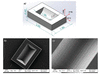Figure 1 Sketch of the planar diamond half-lens with dimensions (in mm) and showing crystallographic directions (a). SEM images of the lens. Overview (b) and magnified profile (c).

### 3. Optical performance of the CRL

A diamond CRL composed of 12 single half-lenses was tested at the Micro Optics Test Bench (Snigirev et al., 2007) at the ID06 beamline of the ESRF. A beam was produced by an in-vacuum undulator, and desired X-ray energies were selected by a cryogenically cooled Si (111) double-crystal monochromator. Effective vertical and horizontal source sizes were 40 µm and 900 µm [full width at half-maximum (FWHM)], respectively. We located the diamond CRL at 56.3 m from the source and mounted it on the motorized stage with all necessary translation/rotation movements, as shown in a sketch of the experimental setup (Fig. 2). The CRL was oriented in such a way as to focus X-radiation in the vertical direction, and an upstream vertical slit size was adjusted to avoid radiation impinging on the CRL outside of its effective aperture of 260 µm (Kohn, 2009). The size of the horizontal slit (1200 µm) used almost corresponded to the length of the CRL. The CRL's effective aperture had been previously measured by scanning with a 10 µm-wide vertical slit while recording the propagated intensity with a silicon pin-diode. The measured value of 260 µm fully co­incided with the theoretical value. The diamond CRL had a calculated imaging distance of 1.5 m at an X-ray photon energy of 8 keV; therefore, we expected to achieve a source demagnification factor of 37 (as a ratio of source-to-lens to imaging distances). Measurements of the CRL's efficiencies and sizes of focal lines were performed using a PCO X-ray camera. This is a CCD camera where a high resolution and a low pixel size (0.73 µm) are achieved by a system based on a thin scintillator and visible photon magnifying optics.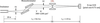Figure 2 Layout for measurements of the CRL's focusing properties at the ID06 beamline.

An image of the focused X-ray beam measured for an imaging distance of 1.53 m is shown in Fig. 3. The focal line is uniform and its vertical size is 2.2 µm (FWHM of the Lorentzian function which fits the intensity distribution). Unfortunately, the measured width of the focal line is twice as large as the predicted value of 1.1 µm (according to the demagnification factor). This can be attributed to a non-ideal lens profile. Indeed, deviation of the real imaging distance from its theoretical value indicates that the real lens radius has a value of 204 ± 1 µm (an average for all the lenses in the CRL). In parallel, we measured the CRL's gain, i.e. the integral intensity in the focal plane divided by the integral intensity from the same area without the lens in the path of the beam as described by Lengeler et al. (1999). A value of 20 was obtained.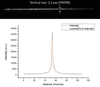Figure 3 Focal line produced by a CRL with 12 diamond planar half-lenses. The vertical size of the image is 2.2 µm (FWHM of the Lorentzian function which fits the intensity distribution) and the horizontal size corresponds to the width of the slit S2.

To measure the profile of a single half-lens, we used the X-ray grating interferometry technique (David et al., 2002; Zanette, 2011; Koch et al., 2016). A grating interferometer is a Talbot-type interferometer with a single line grating of period 10 µm (3 µm-high Ni lines), providing a phase shift of π/2. The grating was mounted on a piezo scanner with the lines oriented horizontally to make the best use of the coherence of the asymmetric source at ID06. It was placed on the optics table (Fig. 4) at 17 cm after a single diamond half-lens which was used as the sample. The X-ray CCD detector was put at the one-quarter Talbot imaging distance of 69 cm from the grating. The monochromator was tuned to select X-rays with energy of 17 keV. Then, two interference patterns were recorded: the first with the sample in the beam, and the second, for reference, with the sample out of the beam. The lens refraction angle α(x) in the direction perpendicular to the grating lines was obtained from the measurement of the displacement of fringes from one image to another. A derivative of the lens profile was then calculated from the formula=, where δ is the refractive index decrement of the lens material (diamond). As a result of the procedure, by integration of the profile derivative, a profile of the lens was reconstructed. We should note that this profile is averaged over the lens width of 150 µm (Fig. 5b). The profile of a single diamond half-lens reconstructed from the interference pattern (black curve) and an ideal parabolic curve with the radius at the parabola apex of 208 µm (blue curve) are presented in Fig. 5(a). A discrepancy between the two curves is also shown (red curve) with multiplication of the y axis by 10. One may see that the amplitude of the profile's deviation lies within the interval ±2.5 µm and has an average period of 50 µm.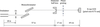Figure 4 Layout of the experiment measuring the diamond lens profile by X-ray Talbot grating interferometry.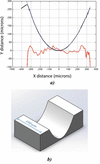Figure 5 (a) Profile of a single diamond half-lens reconstructed from the interference pattern (black curve), and the ideal parabolic curve with the radius at the parabola apex of 208 µm (blue curve). A discrepancy between the two curves is also shown (red curve) with the y axis multiplied by 10. (b) Profile averaged over the lens width of 150 µm.

The sensitivity of the grating interferometer is limited by the period of the phase grating. However, surface imperfections with a period of less than 10 µm may be observed by X-ray radiography, where a sample is exposed to X-rays with registration of the signal passed. At 8 keV, an X-ray image of the single half-lens and intensity variation obtained through the vertical cross section of the image are shown in Fig. 6. The cross section is averaged over a width of 150 µm to match the averaging in the Talbot interferometry experiment. Here we see longitudinal periodic grooves (Fig. 6b) which were introduced by the laser and have an average period of 8 µm.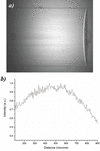Figure 6 X-ray radiography of the single half-lens (a) with its vertical cross-section (b). The cross section is averaged over a width of 150 µm to match the averaging in the Talbot interferometry experiment.

Slope errors can be classified into two categories: figure and finish. Finish errors have high-order spatial frequencies and indicate the roughness of the surface. In the case of single-crystal materials with almost perfect inner structure, parasite X-ray scattering comes from surface imperfections and decreases the gain factor, so can be used to estimate the roughness. The theoretical value of the gain, G, can be calculated as described by Tummler (2000) using the formula G =withwhere A reflects the physical aperture of the lens, Bv is the vertical size of the focused image, Tp is the transmission of the parabolic CRL, N is the number of half-lenses, μ is the linear absorption coefficient, d is the websize of the half-lens, δ is the refractive index decrement, λ is the wavelength of the X-radiation and τ is the r.m.s. surface roughness. If we assume τ = 0.65 ± 0.05 µm in the formula above, we obtain the calculated value of the gain, that corresponds to experimentally obtained value.

Figure errors have low-order frequencies and in other words they are deviations from the overall geometry of the lens. They introduce spherical aberrations and increase the spot size of a focused beam. The maximum figure slope error (Church, 1995) at the simplest level can be calculated as the spot size of the focused beam divided by the imaging distance, and a value of 1.44 µrad can be obtained. For more precision, let us consider figure errors as the sine-modulated deviation d(x) from the ideal parabolic profile (Polikarpov et al., 2015) in the direction normal to the lens surface. Relying on the obtained experimental results, we could presume the presence of two low-order frequencies with periods of deviation T1 and T2. In this case,where d0 is the magnitude of the deviation, L(x) is the arc length of the parabola and x is the coordinate across the optical axis. The arc length of the parabola with the radius of curvature, R, at the parabola apex isFig. 7(a)shows an ideal parabolic lens profile together with the sine-modulated profile with a magnitude of d0 = 2.5 µm and periods of T1 = 8 µm and T2 = 50 µm. The calculated intensity distributions across the optical axis for focusing by the ideal and the distorted (d0 = 2.5 µm and d0 = 2 µm) lens profiles are presented in Fig. 7(b). We assumed here the geometry of the real experiment where a plane wave from a point source has been focused by 12 single half-lenses with radius R = 206 ± 2 µm (the averaged value for interferometry and focusing experiments). Clearly, degradation of the ideal focus is more pronounced as d0 increases. The width of the focal line (FWHM) calculated for d0 = 2.5 µm [red curve in Fig. 7(b)] is larger than the ideal one by 70%. Such a result does not exactly match the experimentally observed blur of the focal line (FWHM degraded by 100%). However, the discrepancy can also be explained by the matrix limitations of the CCD camera, while the camera is incapable of detecting a signal with an accuracy better than 3 pixels (3 pixels = 2.2 µm).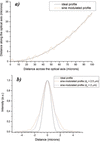Figure 7 (a) Calculated ideal parabolic and sine-modulated (d0 = 2.5 µm, T1 = 8 µm, T2 = 50 µm) lens profiles. (b) Calculated intensity distributions in the image plane for ideal parabolic and sine-modulated (T1 = 8 µm, T2 = 50 µm) lens profiles.

To summarize, this was a simple illustration of the degradation of a CRL's focusing properties with increasing magnitude of figure errors. Such periodic deviations from the ideal profile have a strong and negative influence on the optical properties of the lens in terms of width (and gain) of the focal line. Therefore, this implies an urgent need to carry out lens surface post processing to improve the optical performance. This can be realised by polishing with either an excimer laser or with a focused ion beam. The magnitude d0 has to be reduced, at least, to 0.5% of the lens radius.

### 4. Spectral X-ray glitches

As far as X-ray refractive lenses are made from a single-crystal diamond, depending on the crystallographic orientation of the lens substrate or on the photon energy of the incident-to-lens X-rays, the Bragg law can be satisfied. Owing to Bragg diffraction, the transmitted intensity might be dramatically reduced. This problem is well known in X-ray spectroscopy under high pressure using diamond anvil cells (Chen et al., 2013) and is called a glitch'. To avoid misunderstanding, in our paper we do not consider X-ray glitches in terms of multi-beam diffraction only (Cole et al., 1962; van Zuylen & van der Hoek, 1986; Van Der Laan & Thole, 1988) when a single-crystal is oriented in the X-ray beam in such a way that two or even more sets of crystal planes simultaneously satisfy Bragg's conditions for a single wavelength. In contrast, we would like to demonstrate the existence of the diffraction in the focusing mode of the CRL and to measure the magnitude of the effect.

The setup used for this part of the experiment (Fig. 2) was the same as for the experiments described above, except that a PIN-diode was additionally installed in front of the X-ray CCD. We performed an energy scan with the monochromator in the range 11–11.3 keV while recording transmission through the CRL integral intensity by the PIN diode. The intensity scan with the CRL in the beam was normalized using the scan with no CRL in the beam and is shown in Fig. 8. We observe a large amount of intensity minima where the diffraction conditions were satisfied. We should note that intensity drops are relatively small:takes values from 6% to 10%.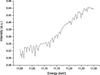Figure 8 Dependence of the signal transmitted through the CRL on the energy of the incident X-rays. This is a relative intensity: a scan with the CRL in the beam was normalized by a scan with no CRL in the beam.

This effect can be explained in the following way. Let us assume that all individual lenses within the diamond CRL are perfectly oriented. By the word `perfectly', we mean that their crystallographic planes [for example (100)] are parallel to each other with an angular accuracy of about FWHM of the rocking curve which is of the order of a few seconds of arc at an energy of 11 keV. However, real lenses are processed from different diamond plates where the angle between (100) planes and the plate's surface may vary within a few degrees. This leads to splitting of the one strong minimum which could be observed for the bulk single-crystal material to a number of individual minima with relatively low intensity drop.

As an addition, we made narrow energy scans in several regions of interest around the X-ray glitches' minima (11.051, 11.062 and 11.075 keV) while recording the focused X-ray beam with the X-ray CCD camera. We have not seen any degradations of the focal spot's size or shape and, predictably, we observed the darkening of the focal spot at the exact energies of the X-ray glitches.

### 5. Conclusion

In the present research we have demonstrated planar refractive lenses manufactured from single-crystal diamond with laser ablation by a Nd:YAG picosecond laser. Compared with the previously tested lenses (Kononenko et al., 2016; Polikarpov et al., 2015), distinctive features of the new lenses are the method of profile processing and, as a result, the lens geometry; such a one-dimensional profile has no limitations neither on the lens aperture and width nor on the profile's depth. Unlike planar lenses cut from a single plate, the new lenses are free from the problem of non-vertical side walls. Additionally, as we mentioned before, single-crystal diamond is a perfect thermo- and radiation-resistant material. The above facts allow such lenses to be installed as pre-focusing and beam-shaping elements at front-end sections of modern X-ray accelerator sources, delivering radiation with no losses even at low-β synchrotron sections, where the beam size still may reach several millimetres.

The lens has been tested in a focusing mode and the obtained focused image was twice the predicted value due to the non-ideal lens profile. A reduced value of the measured gain can be attributed to the roughness of the surface. Based on experimental measurements and theoretical modelling of profile errors, further research is indispensable to reduce figure errors down to the value of 0.5% of the lens radius. Improvement of the profile quality should be done by introducing a correction algorithm to the laser manipulation process. It will take into account a displacement of the laser focus while moving from the edge to the centre of the lens. This should pull the radius error down to a value of 0.5–1%. We also believe that the use of femto-second lasers will significantly reduce aberrations of the lens and, therefore, optical efficiency. As was already shown by Kononenko et al. (2016), femto-second laser ablation is able to produce refractive lenses with smooth surfaces which are free of wavy profiles. Additionally, femto-second lasers provide a surface roughness value of 0.3 µm (r.m.s.). Indeed, this value may be several times reduced by subsequent polishing. According to our estimations, the depth of the damaged layer by laser (which consists partially of the graphitized carbon) may reach 1 µm. The graphite phase should be removed by boiling in hot acid with a subsequent treatment of the lens' surface by mechanical or chemical-assisted mechanical, ion-beam, abrasive liquid or excimer-laser polishing (Ozkan et al., 1997; Chen & Zhang, 2013).

The presence of X-ray spectral glitches was observed by monitoring an intensity transmitted through the CRL. Predictably, the effect arises at certain energies and affects the CRL's gain factor while keeping invariable the transmitted wavefront. As discussed above, the contribution of X-ray glitches can be minimized by manufacturing single refractive lenses from various diamond plates with slightly different crystallographic orientation. In such a case, the intensity drops are relatively small and may be comparable with X-ray glitches from monochromators, which are caused primarily by three- or many-beam cases and have a drop value of 2–3%. To summarize, one can say that X-ray glitches from single-crystal X-ray lenses may only impact spectroscopy or similar experiments whereas the main part of the experiments using refractive optics (focusing, imaging, microscopy and diffraction) should ignore this effect.

### Acknowledgements

The authors express their gratitude to Carsten Detlefs, Pierre Wattecamps and Michael Lyubomirskiy for invaluable contribution to conducting the experiment. The work was supported by the Ministry of Education and Science of the Russian Federation, contract Nos. 14.Y26.31.0002 and 02.G25.31.0086.

### ReferencesJOURNAL OFSYNCHROTRONRADIATION